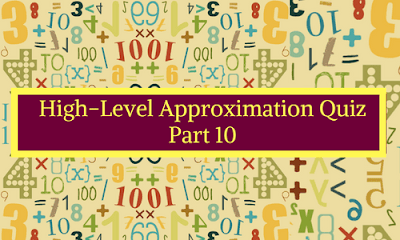# High-Level Approximation Quiz: Part 101. What approximate value should come in the place of question mark (?) in the following question?A.  462
B. 380
C. 250
D. 280
E. 342

2. What approximate value should come in the place of question mark (?) in the following question?A. 24
B. 16
C. 18
D. 12
E. 26

3. What approximate value should come in the place of question mark (?) in the following question?A. 38
B. 28
E. 24
D. 22
E. 32

4. What approximate value should come in the place of question mark (?) in the following question?A. 18055
B. 18405
C. 18205
D. 16405
E. 17805

5. What approximate value should come in the place of question mark (?) in the following question?A. 64
B. 4
C. 16
D. 9
E. 27

6. What approximate value should come in the place of question mark (?) in the following question?A. 9
B. 17
C. 7
D. 27
E. 29

7. What approximate value should come in the place of question mark (?) in the following question?A. 6.5
B. 5
C. 7.5
D. 8
E. 18

8. What approximate value should come in the place of question mark (?) in the following question?A. 8.66
B. 7225
C. 9.21
D. 5625
E. 7.21

9. What approximate value should come in the place of question mark (?) in the following question?A. 8
B. 6
C. 9
D. 11
E. 7

10. What approximate value should come in the place of question mark (?) in the following question?A. 43
B. 33
C. 37
D. 29
E. 39

#### 1. Ans. C.#### 2. Ans. B.#### 3. Ans. B.#### 4. Ans. A.#### 5. Ans. D.#### 6. Ans. C.#### 7. Ans. B.#### 8. Ans. D.#### 9. Ans. E#### 10. Ans. D.